top of pageSearch
• Mani Abedini

# Generative vs Discriminative Learning Algorithms

Updated: Jul 27, 2020

What is the difference between generative and discriminative models?

Generative models explain how the data is generated. The focus is on learning the distribution of data points and associated classes. Whereas, Discriminative models explain how to differentiate (discriminate) classes, the focus in discriminative learning is on searching for decision boundry and separating classes.To formulate the difference; Discriminative models learn the conditional probability of "y" given the "x":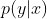Generative models on the other hand learns the joint distribution of "x" and "y".Expanding the definition of conditional probability, the joint distribution is equal to conditional probability of "x: given "y" and marginal probability of "y":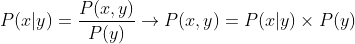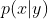means what the distribution of x looks like for a given class "y".or "class prior", suggests the probability of new sample belongs to class "y" regardless of knowing anything about "x".

As we can see, generative models give more insight about data distributions and their boundaries. However, in order to calculate classification probabilities, we need a little of more calculation to do.

How to use a trained (generative or descriminitive) model for classification?

Discriminative models, as their name suggest, are naturally estimate the probability of classification of y given x.However, for generative learning algorithms, once we trained a model from data, we can use Bayesian Theorem to calculate the probability of classification; as follow:Note for binary class (y), there are two outcomes: "y" or "Not y".

For example, to calculate the probability of "x" belongs to class "y=1":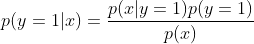We already have the estimates of numerators from data:The denominator can be calculated based on join probability, from formula below: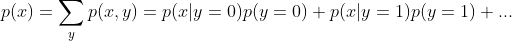All of the terms are given by generative model. Thus, we can predict the probability of class "y=1" for given "x".

Let's illustrate the difference between Generative and Discriminative classification models with a super simple example.

Suppose we have the following data:

`(x,y)={(0,0),(0,1),(0,1),(1,0),(1,1),(1,1),(1,0)}`

Based on discriminative approach, P(y|x) for all y are as follow:

```+-----+-----+-----+
|     | y=0 | y=1 |
+-----+-----+-----+
| x=0 | 1/3 | 2/3 |
+-----+-----+-----+
| x=1 | 1/2 | 1/2 |
+-----+-----+-----+```

In this case the probability of y=1 if x=0 is

`P(y=1|x=0) = 2/3`

Based on generative approach, P(x,y) table is as follow:

```+-----+-----+-----+
|     | y=0 | y=1 |
+-----+-----+-----+
| x=0 | 1/7 | 2/7 |
+-----+-----+-----+
| x=1 | 2/7 | 2/7 |
+-----+-----+-----+```

In this case the probability of having x=0 and y=1 at the same time is: 2/7 (some insight about the class x and y distribution).

In terms of classification, for the given x=0, the probability classification of y=1 is:

`P(y=1|x=0) = P(x=0, y=1) / P(x=0) = 2/3`

Summary:

For classification purpose, both models use conditional probability, but in general it seems Discriminative models are generally more accurate than Generative models, because discriminative learning algorithm is focus on finding the decision boundary (classification task) while generative learning algorithm is more focus on modeling the data; which can be used for classification, but also, we can generate synthetic data from the learned generative model as well.

Generative classifier such as: Naive Bayes, Gaussian mixture model, Generative Adversarial Networks (GAN), Variational Autoencoders (VAE).

Discriminative classifier such as: Logistic Regression, KNN, SVM, Neural Network.